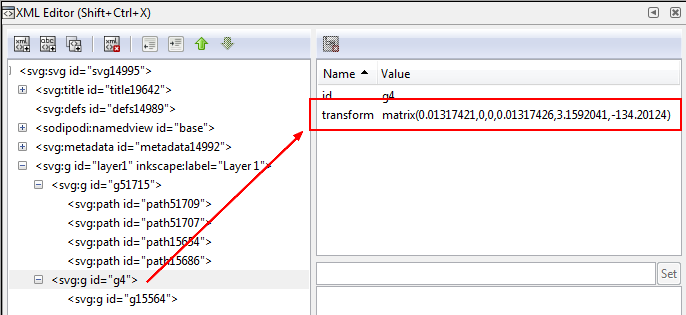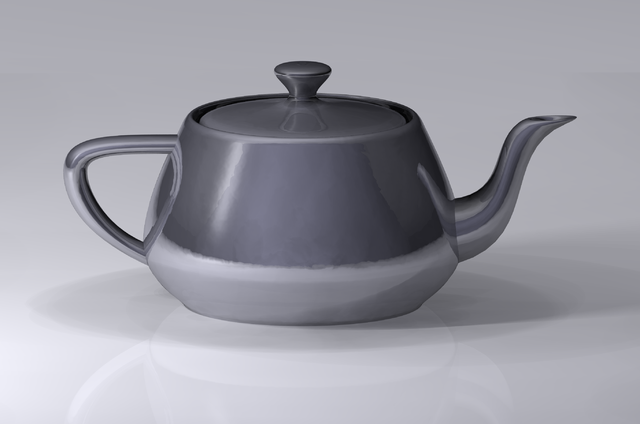# Geometry - Transformation Matrix

A geometric transformation can be represented by a matrix.

THE advantage of using transformation matrices is that cumulative transformations can be described by simply multiplying the matrices that describe each individual transformation.

To represent affine transformations with matrices, homogeneous coordinates are used. This means representing a 2-vector (x, y) as a 3-vector (x, y, 1), and similarly for higher dimensions.

## Structure for a 2D

A 3 × 3 matrix that describes how an object is to be transformed in a 2D plane where:

In non-matrix form (linear), we have the transformation: $$\begin{array}{rrl} x' & = & ax + cy + e \\ y' & = & bx + dy + f \\ \end{array}$$

which is equivalent in matrix form to

$$\begin{bmatrix} x' \\ y' \\ 1 \end{bmatrix} = \begin{bmatrix} a & c & e \\ b & d & f \\ 0 & 0 & 1 \end{bmatrix} \begin{bmatrix} x \\ y \\ 1 \end{bmatrix} = \begin{bmatrix} ax + cy + e\\ bx + dy + f \\ 1 \end{bmatrix}$$

where (x', y') is the new coordinate of a point at (x, y).

For:

• a translation: e is magnitude of a translation in the x direction and f is magnitude of a translation in the y direction.
• scaling, a and d are the scale factors for the x and y directions, respectively.
• a pure rotation, a = d = sin(theta) and b = -c = cos(theta) where theta is the angle of the desired rotation.
• skewing, c and b control skewing parallel to the x and y axes, respectively.

## Documentation / Reference

Discover MoreGeometry - Scaling

Scaling is a transformation that is generally applied by the transformation matrix See also: The functional form becomes the following matrix. Using the standard transformation matrix notation,...Geometry - Skewing (Shearing)

Shear is a transform that rotates one axis so that the axes are no longer perpendicular. It means offsetting the coordinates along one or two axes based on the distance along the remaining axis. Under...Geometry - Transformation

Transformation of geometric objects. A geometric transformation is represented by a transformation matrix. Transformations that keep the origin fixed are linear including: , ...A translation is a special type of transformation matrix. See . The functional form becomes: In Svg, the transform matrix is implemented with the matrix function. A translation would be matrix(1...Inkscape - Object Transformation

Inkscape stores an object's transformation internally as a transformation matrix (which can be seen and modified with the XML Editor). A transformation matrix is always defined with respect to some...Viz - Scene Graph - Graphical World (Tree Data Structure)

A scene graph is a collection of nodes in a tree structure. It's equivalent to a document in xml The node of the tree are: a graphical object for the leaf a compound object such as: or a group...